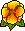## Maxwell and Lamé Eigenvalues on Polyhedra

Martin Costabel, Monique DaugeIn a convex polyhedron, a part of the Lamé eigenvalues with hard simple support boundary conditions does not depend on the Lamé coefficients and coincide with the Maxwell eigenvalues. The other eigenvalues depend linearly on a parameter \$s\$ linked to the Lamé coefficients and the associated eigenmodes are the gradients of the Laplace-Dirichlet eigenfunctions. In a non-convex polyhedron, such a splitting of the spectrum disappears partly or completely, in relation with the non-\$H^2\$ singularities of the Laplace-Dirichlet eigenfunctions. From the Maxwell equations point of view, this means that in a non-convex polyhedron, the spectrum cannot be approximated by finite element methods using \$H^1\$ elements. Similar properties hold in polygons. We give numerical results for two L-shaped domains.Preprint IRMAR 98-08 (Mar. 98)

Published in Mathematical Methods in the Applied Sciences 22 (1999) 243-258.Fichier pdf (236 k) --- Fichier postscript (269 k)